Courses

# Notes | EduRev

## JEE : Notes | EduRev

The document Notes | EduRev is a part of the JEE Course Mock Test Series for JEE Main & Advanced 2022.
All you need of JEE at this link: JEE

Question 1: The density of a 3M sodium thiosulphate solution is 1.25 gm cm–3. Calculate
(i) the molalities of Na+ and S2O32–  ions
(ii) percentage of weight of solution
(iii) mol-fraction of sodium thiosulphate.
Solution: 3 M Na2S2O3 (Sodium thiosulphate) solution means
3 moles Na2S2O3 is present in 1 L or, 1000 ml solution
Wt. of solute Na2S2O3 = 3×158
wt. of solution = v × d
= 1000 ml × 1.25 gm/ml
= 1000 × 1.25 gm
Wt. of solvent = (1000 × 1.25 – 3 ×158) gm H2O
Molality = no. of moles of solute per 1000 gm solvent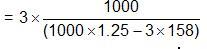= 3.865 mol kg–1 solvent
Now, Na2S2O3  2Na+ +S2O3-
(a) Hence molality of Na+ = 2 ×3.865 mol kg-1
= 7.73 mol kg–1
Hence molality  = 1× 3.865 mol kg–1
= 3.865 mole/kg
(b) % of wt. of solution
1 L i.e. 1000 ml solution containing 3 moles Na2S2O3
1000 × 1.25 gm solution containing 3 ×158 gm Na2S2O3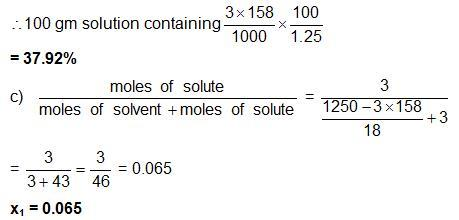Question 2: 8.0575 × 10–2 kg of glauber’s salt (Na2SO4.10H2O) is dissolved in water to obtain 1 dmof a solution of density 1077.2 kgm–3. Calculate the molality, molarity and mole fraction of Na2SO4 in the solution.
Solution:
Wt. of Glauber’s satt = w1 = 8.0575 × 10–2 kg
= 80.575 gm
density of solution = d = 1077.2 kg m–3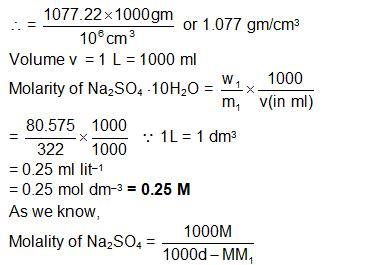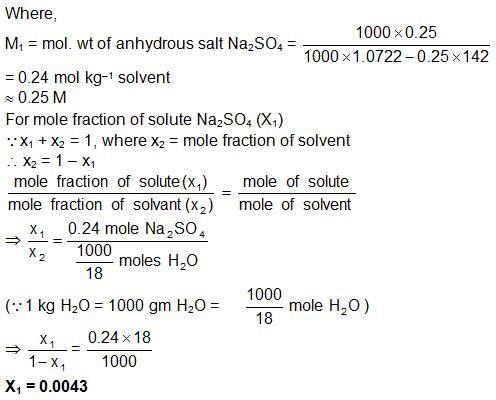Question 3: A liquid mixture of benzene (mole fraction 0.33) and toluene (mole fraction 0.67) is taken. What will be the composition of vapours over the liquid mixture? This vapours is collected, condensed to a liquid and then allowed to evaporate so as to come into equilibrium with its vapour. What is composition of new vapour [Temperature = 20°C]. Given is vapour pressure of pure benzene = 75 torr at 20°C and vapour pressure of pure toluene = 22 torr)
Solution:
P= 75 ×0.33 + 22 0.67 = 24.75 + 14.74 = 39.49 torr
The composition of the vapour in mole fraction units can be obtained by using Dalton’s law. In vapour phase we have;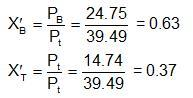Again
Now the mole fraction of benzene and toluene in liquid are 0.63 and 0.37 respectively. Therefore, their vapour pressures are given by
P= 0.63 × 75 + 0.37 × 22 = 47.25 + 8.14 = 55.39 torr
Now we can calculate the mole fractions in vapour phase to be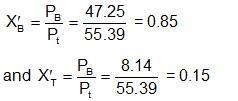Offer running on EduRev: Apply code STAYHOME200 to get INR 200 off on our premium plan EduRev Infinity!

## Mock Test Series for JEE Main & Advanced 2022

2 videos|258 docs|160 tests

,

,

,

,

,

,

,

,

,

,

,

,

,

,

,

,

,

,

,

,

,

;